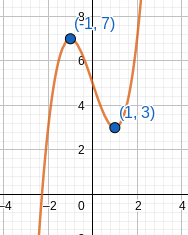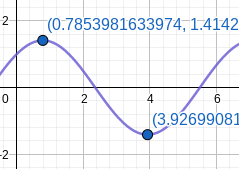# Given the functions below, use the transition points and the first/second derivative sign...

## Question:

Given the functions below, use the transition points and the first/second derivative sign combinations on the intervals corresponding to the transition points to sketch the graph. Use GeoGebra to check your answer.

a. {eq}f(x) = x^3 - 3x + 5 {/eq}

b. g(x) = sin(x) + cos(x) on {eq}[0, 2\pi] {/eq}

## Find and Classify Critical Points:

The critical points of the function {eq}f(x) {/eq} are the zeros of the first derivative. That is, the solutions to the equation {eq}f'(x) = 0 {/eq}. The second derivative test allows us to classify the critical points. To implement this test, we evaluate the second derivative at each critical point. If the value of the second derivative is negative, the critical point corresponds to a maximum. If the value of the second derivative is positive, the critical point corresponds to a minimum.

a. {eq}f(x) = x^3 - 3x + 5 {/eq}

We must first find the critical points of the function. To do this, we find the equation of the first derivative, set it equal to {eq}0 {/eq} and solve for {eq}x {/eq}.

\begin{align*} f(x) &= x^3 - 3x + 5 \\ f'(x) &= 3x^2 - 3 \\ 0 &= 3x^2 - 3 \\ x^2 &= 1 \\ x &= \pm 1 \end{align*}

The critical points of the function are {eq}x = -1 {/eq} and {eq}x = 1 {/eq}.

To classify the critical points, we use the second derivative test. We evaluate the second derivative at each critical point.

\begin{align*} f(x) &= x^3 - 3x + 5 \\ f'(x) &= 3x^2 - 3 \\ f''(x) &= 6x \\ f''(-1) &= 6(-1) = -6 \\ f''(1) &= 6(1) = 6 \end{align*}

From the values of the second derivative, we determine the function has a maximum at {eq}x = -1 {/eq} and a minimum at {eq}x = 1 {/eq}.

This means the graph is increasing to the left of {eq}x = -1 {/eq} and decreasing to the right, until the point {eq}x = 1 {/eq} when the graph then begins to increase again.b. {eq}f(x) = \sin(x) + \cos(x) {/eq} on {eq}[0, 2\pi] {/eq}.

We once again begin by finding the equation of the first derivative. Then, we set the derivative equal to {eq}0 {/eq} and solve for the values of {eq}x {/eq} that satisfy the equation.

\begin{align*} f(x) &= \sin(x) + \cos(x) \\ f'(x) &= \cos(x) - \sin(x) \\ 0 &= \cos(x) - \sin(x) \\ \cos(x) &= \sin(x) \\ x &= \frac{\pi}{4}, \frac{5\pi}{4} \end{align*}

The critical points of the function are {eq}x = \frac{\pi}{4} {/eq} and {eq}x = \frac{5\pi}{4} {/eq}.

To classify the critical points, we use the second derivative test. This means we evaluate the second derivative at each critical point.

\begin{align*} f(x) &= \sin(x) + \cos(x) \\ f'(x) &= \cos(x) - \sin(x) \\ f''(x) &= -\sin(x) - \cos(x) \\ f''(\frac{\pi}{4}) &= -\sin(\frac{\pi}{4}) - \cos(\frac{\pi}{4}) = -\sqrt2 \\ f''(\frac{5\pi}{4}) &= -\sin(\frac{5\pi}{4}) - \cos(\frac{5\pi}{4}) = \sqrt2 \end{align*}

From the second derivative test, we determine there is a maximum at {eq}x = \frac{\pi}{4} {/eq} and a minimum at {eq}x = \frac{5\pi}{4} {/eq}.

With this information, we determine the function is increasing to the left of {eq}x = \frac{\pi}{4} {/eq}, decreasing from {eq}x = \frac{\pi}{4} {/eq} to {eq}x = \frac{5\pi}{4} {/eq} and increasing to the right of {eq}x = \frac{5\pi}{4} {/eq}.